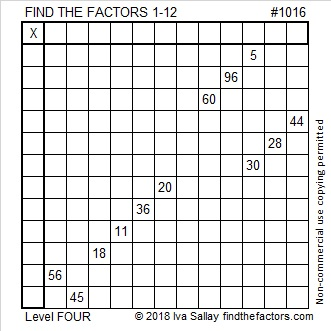# 1016 and Level 4

If you’ve never tried a level 4 puzzle before, this is a great one to try. Of its twelve clues, eight have only one factor pair in which both factors are from 1 to 12. You should easily be able to place the factors for those eight clues. Since each factor from 1 to 12 must appear exactly one time in the first column and the top row, the factors from those eight clues will eliminate some of the possible factors of the other four clues. Don’t be afraid to give this puzzle a try!Print the puzzles or type the solution in this excel file: 12 factors 1012-1018

Here are some reasons why 1016 is an interesting number:

1016 is the sum of seven consecutive powers of two:
2⁹ + 2⁸ + 2⁷ + 2⁶ + 2⁵ + 2⁴ + 2³ = 1016
I know that’s true because 1016 is 1111111000 in BASE 2

1016 is a palindrome in a couple of bases as well:
It’s 13031 in BASE 5 because 1(5⁴) + 3(5³) + 0(5²) + 3(5¹) + 1(5⁰) = 1016
161 in BASE 29 because 1(29²) + 6(29¹) + 1(29⁰) = 1016

1016 is divisible by 2 because 6 is even.
1016 is divisible by 4 because 16 is divisible by 4. (And also because 6 is divisible by 2 but NOT by 4 and 1 is odd.)
1016 is divisible by 8 because 016 is divisible by 8. (And because 16 is divisible by 8 and 0 is an even number.
1016 is NOT divisible by 16 because 016 is divisible by 16 and 1 is an odd number.

• 1016 is a composite number.
• Prime factorization: 1016 = 2 × 2 × 2 × 127, which can be written 1016 = 2³ × 127
• The exponents in the prime factorization are 3 and 1. Adding one to each and multiplying we get (3 + 1)(1 + 1) = 4 × 2 = 8. Therefore 1016 has exactly 8 factors.
• Factors of 1016: 1, 2, 4, 8, 127, 254, 508, 1016
• Factor pairs: 1016 = 1 × 1016, 2 × 508, 4 × 254, or 8 × 127
• Taking the factor pair with the largest square number factor, we get √1016 = (√4)(√254) = 2√254 ≈ 31.87475